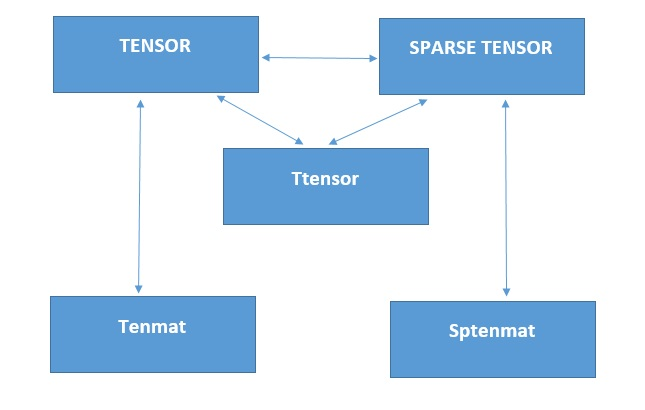# Sparse Tensor

Back to glossary
Python offers an inbuilt library called numpy to manipulate multi-dimensional arrays. The organization and use of this library is a primary requirement for developing the pytensor library.Sptensor is a class that represents the sparse tensor. A sparse tensor is a dataset in which most of the entries are zero, one such example would be a large diagonal matrix. (which has many zero elements). It does not store the whole values of the tensor object but stores the non-zero values and the corresponding coordinates of them. Sparse tensor storage formats allow us to only store non-zero values thereby reducing storage requirements and eliminating unnecessary silent computations involving zero values. Here are its main attributes:
• vals (numpy.ndarray) A 1-dimensional array of non-zero values of the sparse tensor.
• subs (numpy.ndarray) A 2-dimensional array of coordinates of the values in vals.
• shape(tuple)
The shape of the sparse tensor.
• func(binary operator) This function is used to construct the sparse tensor as an accumulator.
On top of that, its main functions are:
• __init__(self, subs, vals, shape = None, func=sum.__call__) Consturctor for the sptensor class. subs and vals (numpy.ndarray) or (list) are coordinates and values of the sptensor.
• tondarray(self) This function returns a numpy. ndarray object that has the same values with the sptensor.
• permute(self, order) By applying this function it will return the sptensor object that is permuted by the given order (list).
• ipermute(self, order) Returns the sptensor object that is permuted by the inverse of the given order (list).
• copy(self) Returns the copied sptensor object of the sptensor.
• totensor(self) Returns the tensor object that has the same values with the sptensor.
• nnz(self) Returns the number of non-zero elements in the sptensor.
• ndims(self) Returns the number of dimensions of the tensor.
• dimsize(self, ind)
• Returns the size of the specified dimension. Same as shape[ind].